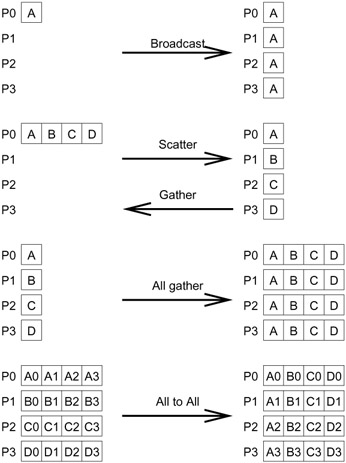# 9.5 More on Collective Operations

## 9.5 More on Collective Operations

One of the strengths of MPI is its collection of scalable collective communication and computation routines. Figure 9.9 shows the capabilities of some of the most important collective communication routines. To illustrate their utility, we consider a simple example.Figure 9.9: Schematic representation of collective data movement in MPI.

Suppose we want to gather the names of all of the nodes that our program is running on, and we want all MPI processes to have this list of names. This is an easy task with MPI_Allgather:

`     char my_hostname[MAX_LEN], all_names[MAX_PROCS][MAX_LEN];     MPI_Allgather( my_hostname, MAX_LEN, MPI_CHAR,                    all_names, MAX_LEN, MPI_CHAR, MPI_COMM_WORLD ); `

This code assumes that no hostname is longer than MAX_LEN characters (including the trailing null). A better code would check this fact:

`     char my_hostname[MAX_LEN], all_names[MAX_PROCS][MAX_LEN];     int my_name_len, max_name_len;     ...     my_name_len = strlen(my_hostname) + 1; `

`     MPI_Allreduce( &my_name_len, &max_name_len, 1, MPI_INT, MPI_MAX,                    MPI_COMM_WORLD );     if (max_name_len > MAX_LEN) {         printf ( "Error: names too long (%d)\n", max_name_len ); `

`     }     MPI_Allgather( my_hostname, MAX_LEN, MPI_CHAR,                    all_names, MAX_LEN, MPI_CHAR, MPI_COMM_WORLD ); `

Both of these approaches move more data than necessary, however. An even better approach is to first gather the size of each processor's name and then gather exactly the number of characters needed from each processor. This uses the "v" (for vector) version of the allgather routine, MPI_Allgatherv, as shown in Figure 9.10. The array all_lens is used to hold the length of the name of the process with rank i in the ith location. From this information, the array displs is calculated, where the ith element is the offset into the character array all_names where the name for the process with rank i begins.`     int all_lens[MAX_PROCS], displs[MAX_PROCS], totlen;     char all_names[MAX_NAMES];     ...     /* Gather the names lengths from all of the processes */     mylen = strlen(my_hostname) + 1;  /* Include the trailing null */     MPI_Allgather( &mylen, 1, MPI_INT, all_lens, 1, MPI_INT,                    MPI_COMM_WORLD );     /* Compute the displacement (displs) of each string in the        result array all-names and total length of all strings */     totlen = all_lens[size-1];     for (i=0; i<size-1; i++) {         displs[i+1] = displs[i] + all_lens[i];         totlen      += all_lens[i];     }     all_names = (char *)malloc( totlen );     if (!all names) MPI_Abort( MPI_COMM_WORLD, 1 );     /* Gather the names from each process, where the name from        process i is all_lens[i] long and is placed into        all names[displs[i]] */     MPI_Allgatherv( my_hostname, mylen, MPI_CHAR,                     all_names, all_lens, displs, MPI_CHAR,                     MPI_COMM_WORLD );     /* Hostname for the jth process is &all names[displs[j]] */ `Figure 9.10: Using MPI_Allgather and MPI_Allgatherv.

This example provides a different way to accomplish the action of the example in Section 8.3. Many parallel codes can be written with MPI collective routines instead of MPI point-to-point communication; such codes often have a simpler logical structure and can benefit from scalable implementations of the collective communications routines.Beowulf Cluster Computing With Linux 2003
ISBN: N/A
EAN: N/A
Year: 2005
Pages: 198